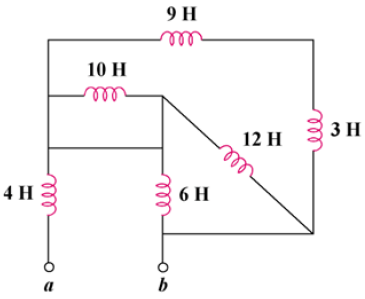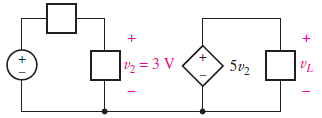# SSC JE Electrical Important MCQ Part 29

SSC JE Electrical Important MCQ PDF Part 29

1.The equivalent capacitance seen between terminals a and b of the circuit in Fig. isA. 4 μF

B. 30 μF

C. 20 μF

D. 60 μF

(The 20-μF and 5-μF capacitors are in series; their equivalent capacitance is 20×5/20+5=4 μF

This 4-μF capacitor is in parallel with the 6-μF and 20-μF capacitors; their combined capacitance is 4 + 6 + 20 = 30 μF

This 30-μF capacitor is in series with the 60-μF capacitor. Hence, the equivalent capacitance for the entire circuit is

Ceq = 30×60/30 + 60 = 20 μF)

2.The equivalent inductance looking into the terminals of the circuit in Fig. isA. 7H

B. 4H

C. 3H

D. 2H3.In the circuit of Fig. if υ2 is known to be 3 V, find υLA. 15 V

B. 5 V

C. 3 V

D. 18 V

Answer : A (v2 = 3 V,vL = 5v2 = 5×3=15 V)

4.What will be the peak power circuit shown in Fig.A. 2 MW

B. 20 MW

C. 200 MW

D. 2000 MW

Answer : B (P = E/t=400 mJ/20 ns= 400×10-3/20×10-9 = 20 MW)

5.You have to replace 1500 Ω resistor in radio. You have no 1500 Ω resistor but have several 1000 Ω ones which you would connect

A. two in parallel

B. two in parallel and one in series

C. three in series

D. three in parallel

Answer : B (If we connect two 1000 Ω resistors in parallel

Then the total resistance R=1000*1000/2000=500 Ω.

Now, if we connect this parallel combination of two 1000 Ω resistance in series with another 1000 Ω resistance

Then the total resistance R=1000+500=1500 Ω.

Hence, we need three 1000 Ω resistors with the connection of two in parallel and one in series.)

Electrical One Year Test Series Pass-Daily Test Plus Weekly Full Test

6.Which of the following material has nearly zero temperature co-efficient of resistance

A. Manganin

B. Porcelain

C. Carbon

D. Copper

Answer : A (Manganin ( CuMnNi) Composition-Copper (Cu): 86%,Manganese (Mn): 12%,Nickel (Ni): 2%,This alloy has a low temperature coefficient of resistance (nearly zero temperature co-efficient of resistance))

7.A current of 16 amperes divides between two branches in parallel of resistances 8 ohms and 12 ohms respectively. The current in each branch is

A. 6.4 A, 6.9 A

B. 6.4 A, 9.6 A

C. 4.6 A, 6.9 A

D. 4.6 A, 9.6 A

(Given that,Current I = 16 A,First resistance R1= 8 Ω,Second resistance R2=12 Ω

The Current in 8 ohm,I1=R2*I/R1+R2=12*16/20=9.6 A

Now, The current in 12 ohm I2=I-I1=16-9.6=6.4 A)

8.Four wires of same material, the same cross-sectional area and the same length when connected in parallel give a resistance of 0.25 Ω. If the same four wires are connected is series the effective resistance will be

A. 1 Ω

B. 2 Ω

C. 3 Ω

D. 4 Ω

Answer : D (Given that,Four wires of same material, the same cross-section area and the same length .

So, resistance of each wire is R

Equivalent resistance in parallel Rp=0.25 Ω

Now, four wires is connected in parallel

1/Rp=1/R1+1/R2+1/R3+1/R4

1/Rp =1/R + 1/R+1/R+1/R

1/0.25. =4/R

R=1 Ω

So, resistance of each wire is 1 ohm

Now, four wires is connected in series then

The equivalent resistance

Rs=R1+R2+R3+R4

Rs=1+1+1+1=4 Ω)

9.The resistance of a parallel circuit consisting of two branches is 12 ohms. If the resistance of one branch is 18 ohms, what is the resistance of the other

A. 18 Ω

B. 36 Ω

C. 48 Ω

D. 64 Ω

(1/Rp= 1/R1 + 1/R2

1/12 = 1/18 + 1/R2

1/12-1/18 = 1/R2

3-2/36 = 1/R2

1/36 = 1/R2

R2=36 Ω)

10.An instrument which detects electric current is known as

A. voltmeter

B. rheostat

C. wattmeter

D. galvanometer

Answer : D (Galvanometer-A galvanometer is an electromechanical instrument used for detecting and indicating an electric current.

Galvanometer applications-It is used for detecting the direction of current flows in the circuit. It also determines the null point of the circuit. The null point means the situation in which no current flows through the circuit

It is used for measuring the current.

The voltage between any two points of the circuit is also determined through galvanometer)

Electrical One Year Test Series Pass-Daily Test Plus Weekly Full Test

11.The resistance of a copper wire 200 m long is 21 Ω. If its thickness (diameter) is 0.44 mm, its specific resistance is around

A. 1.2 x 10-8 Ω-m

B. 1.4 x 10-8 Ω-m

C. 1.6 x 10-8 Ω-m

D. 1.8 x 10-8 Ω-m

(R=p×L/A

p=R×A/L

A = (π/4) × D2.

p=R×(π × D2)/4L

=21×(3.14 × 0.000442)/4×200

=1.6 x 10-8 Ω-m)

12.Conductance is reciprocal of

A. resistance

B. inductance

C. reluctance

D. capacitance

Answer : A ( The unit of electric resistance is ohm (Ω) R = V / I

where

R = resistance (ohm, Ω)

V = electrical potential (volts)

I = current flow (ampere)

The reciprocal of resistance is conductance

G = 1 / R

where

G = conductance (siemens, S))

13.The S.I. unit of power is

A. Henry

B. coulomb

C. watt

D. watt-hour

Answer : C (Power-Power, in general, is defined as the rate at which energy is transferred or converted or the rate of doing work.P = W / t.

SI Unit of Power-Watt ,1 watt = 1 J/s = 1 kg-m2/s3

Other Power Units-Some of the common power units include ergs per second (erg/s), foot-pounds per minute, dBm, food calories per hour or kilocalories per hour, horsepower (hp), BTU per hour (BTU/h))

14.Electric pressure is also called

A. resistance

B. power

C. voltage

D. energy

Answer : C (Voltage-Voltage, electric potential difference, electric pressure,electromotive force or electric tension is the difference in electric potential between two points.V = W/A or V=IR ,SI Unit of Voltage-Volt)

15.In a purely resistive impedance, there is

A. A net capacitance

B. A net inductance

C. Zero resistance

D. Zero reactance

Answer : D (In electric and electronic systems, reactance is the opposition of a circuit element to the flow of current due to that element’s inductance or capacitance.In a purely resistive impedance there is no inductance or capacitance, So Zero reactance)

Electrical One Year Test Series Pass-Daily Test Plus Weekly Full Test

16.In a power supply, resistors are sometimes connected in series with the diodes in order to

A. Increase the current output

B. Protect the diodes against surge currents

C. Help the diodes discharge

D. Regulate the output voltage

Answer : B (Current limiting resistor used to protect the diodes from surge currents)

17.Semi-controlled devices which have uncontrolled turn on and turn off are

A. Diode, SIT, MCT

B. BJT, IGBT, MOSFET

C. SIT, MCT

D. Diode

Answer : D ( Diode is technically an uncontrolled device (uncontrolled switch). Its switching depends on the applied load voltage itself. It will turn on when the applied voltage is greater than the threshold voltage (no matter what current flows).BJT, MOSFET, IGBT, SIT, MCT are turned on and off by the application of control signals)

18.Retentively is also known as _____

A. Retained reluctance

B. Residual magnetism

C. Residual inductance

D. None of these

Answer : B ( Residual magnetism is defined as the amount of magnetization left behind after removing the external magnetic field from the circuit.This magnetism is alsocalled Retentivity of the material)

19.Dissipation factor (tan δ) of a capacitance is measured by which bridge

A. Anderson Bridge

B. Hay Bridge

C. Schering Bridge

D. Wilms Bridge

Answer : C (The Schering bridge use for measuring the capacitance of the capacitor, dissipation factor, properties of an insulator, capacitor bushing, insulating oil and other insulating materials)

20.The armature in an electrical machine is ___________

A. Always a stator

B. Always a rotor

C. Either a stator or rotor

D. Always a stator in a motor and rotor in a generator

Answer : C (In dc machine, armature is a rotor and for synchronous machine armature is a stator. Therefore armature in an electrical machine is either a stator or rotor)